# Course:CPSC522/Conflict-Driven Clause Learning for the Boolean Satisfiability Problem

Jump to navigation Jump to search

## Conflict-Driven Clause Learning for the Boolean Satisfiability Problem

We discuss the development of conflict-driven clause learning (CDCL) in the context of the Davis-Putnam-Logemann-Loveland (DPLL) algorithm for SAT solving.

Principal Author: Carl Kwan

## Abstract

Boolean satisfiability (SAT) was the first decision problem to be proven $NP$-complete. Despite lack of a known tractable algorithm, many practical problems throughout automated reasoning are solved via reduction to SAT. Most modern SAT solvers instead implement some procedure based on the Davis-Putnam-Logemann-Loveland (DPLL) algorithm. In particular, the inspired conflict-driven clause learning (CDCL) algorithm has found explicit success in the SAT solving community and applications.

In this article, we discuss the seminal paper “A Machine Program for Theorem-Proving” which introduces the DPLL algorithm and the more recent paper “Using CSP Look-Back Techniques to Solve Real-World SAT Instances” which introduces CDCL - the improved sequal to DPLL.

### Builds On

It is assumed that the reader is familiar with predicate calculus and possesses some background in the theory of computation. Mathematical maturity is also beneficial but not imperative.

### Related Pages

SAT solvers have a large role to play in logic programming and its sub-paradigms where the satisfiability of conjunctions of constraints is an important question. AI planning and scheduling were among the first successful applications of SAT solvers. Outside of AI, applications of SAT solving include circuit design, model checking, automatic test-pattern generation, bioinformatics, and theorem proving.

## Content

### SAT

Consider a formula over a Boolean algebra, say,

$a\land \lnot a$or

$a\land (\lnot a\lor b\lor \lnot c)\land (\lnot b\lor \lnot c)\land (b\lor \lnot d).$It is clear that the former formula will always evaluate to false independently of the truth assignments of $a$. But what about the latter formula? Does it have an assignment such that it will evaluate to true? This is the Boolean satisfiability problem.

Definition (SAT). Given a formula over a Boolean algebra, is there a truth assignment such that it is satisfied?

Occasionally, a restriction is made that the provided formula is in conjunctive normal form (CNF), ie. the form

$(x_{11}\lor x_{12}\lor \cdots \lor x_{1n})\land \cdots \land (x_{m1}\lor \cdots \lor x_{mk})$where each $x_{ij}$is a literal. We say a formula is in $n$CNF if it is CNF and each clause has $n$literals. SAT is sometimes posed as the equivalent 3SAT problem.

Definition (3SAT). Given a formula over a Boolean algebra in 3CNF, is there an assignment such that such that is satisfied?

Note that 3SAT is already an instance of SAT and it is a common excercise to show SAT can be reduced to 3SAT.

In the early 1970’s, Stephen Cook and Leonid Levin both independently discovered and proved SAT was $NP$-complete. However, notions of satisfiablity date back to Aristotle’s discovery of logic and, since then, heuristics have been developed to combat the problem. A particular successful (and more recent) algorithm is the namesake of its pioneers: Martin Davis, Hilary Putnam, George Logemann, and Donald W. Loveland.

### DPLL

In 1960, Davis and Putnam published a algorithm for SAT which was refined two years later by Davis along with Logemann and Loveland. The original motivations of Davis et al. for the two papers is towards an automated theorem prover for quantification theory. Moreover, the majority of the DPLL algorithm (the portion occasionally referred to as the DP algorithm) is already set out in "A Computing Procedure for Quantification Theory" and, instead, "A Machine Program for Theorem Proving" serves as a (much shorter) discussion regarding the programming implementation of DP. However, the later paper is sufficiently significant for its modifications - especially its space-saving techniques - that it would prove unbecoming to not discuss it in this article. Thus we primarily reference the second paper for the purposes of this article.

"A Machine Program for Theorem Proving" begins with a pointer to the original DP algorithm and then proceeds to discuss storage techniques for their implementation. The paper also introduces the notion of deleting literals and eliminating clauses. Finally, it concludes with some comments on results pertaining to feasibility and space-efficiency.

For pedagogical purposes, we begin by introducing a potential approach to SAT with a quick and simple example. Then we discuss the DPLL algorithm along with the refinements of the second paper. Lastly, we consider the results and performance of DPLL as outlined in the paper in its historical context.

#### Naïve Approach

From here on, we suppose any formula is provided in CNF.

First, it will prove enlightening to consider a naïve approach to SAT, ie. simply assign variables with arbitrary truth values until a satisfying assignment is found or if there are no other cases. Visually, this can be represented with a tree. For example, suppose the formula of interest is

$(a\lor b)\land (a\lor \lnot b)$in which case, this process can be viewed as so: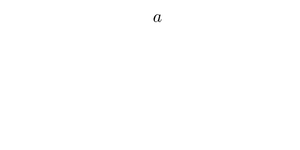Original formula: $(a\lor b)\land (a\lor \lnot b)$Current formula: $(a\lor b)\land (a\lor \lnot b)$Start with a branching variable.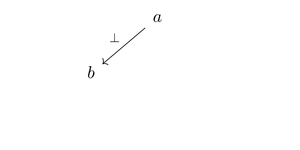Original formula: $(a\lor b)\land (a\lor \lnot b)$Current formula: $(\bot \lor b)\land (\bot \lor \lnot b)$Pick an arbitrary value for the branching variable.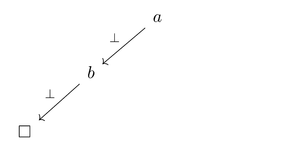Original formula: $(a\lor b)\land (a\lor \lnot b)$Current formula: $(\bot \lor \bot )\land (\bot \lor \top )$Pick an arbitrary value for another variable.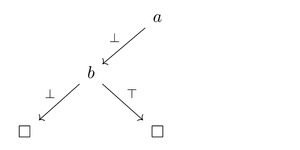Original formula: $(a\lor b)\land (a\lor \lnot b)$Current formula: $(\bot \lor \top )\land (\bot \lor \bot )$If a contradiction occurs, backtrack to the last variable and pick another value.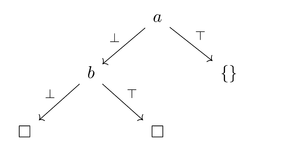Original formula: $(a\lor b)\land (a\lor \lnot b)$Current formula: $(\top \lor b)\land (\top \lor \lnot b)$Repeat until sat (or until the search space has been exhausted)

Here, the box $\Box$denotes an unsatisfactory assignment (unsat) and the empty clause $\{\}$denotes a satisfactory assignment (sat). In the example, the initial choice of $a=\bot$was a poor one in that the path to the solution is the longest possible. It is clear that the naïve approach has exponential time complexity. While DPLL remains exponential, there remains small rules to improve performance.

#### Unit Propagation

There are some very obvious immediate improvements that can be made. For example, if an atom and its negation both appear as one-literal clauses, then the formula is immediately unsat, ie. $a\land \lnot a\land \cdots \equiv \bot .$Now suppose an atom appears as a one-literal clause. Then that atom must be assigned true and any clause containing it is immediately satisfied. However, any negation of the atom will be false. In particular, we may ignore any clauses containing the atom and any instance of the negation of the atom will otherwise have no bearing on the satisfiability of the formula. For example,

$a\land (x_{1}\lor x_{2}\lor a)\land (x_{3}\lor \lnot a)\iff \top \land (x_{1}\lor x_{2}\lor \top )\land (x_{3}\lor \bot )\iff x_{3}\lor \bot \iff x_{3}.$The same applies if a negation of an atom appears as a one-literal clause. A one-literal clause is also called a unit. The process of eliminating the clauses containing the atom (or its negation) and instances of the negation (or its atom, respectively) is called unit propagation.

Algorithm UnitPropagation

1 # Given a formula f in CNF, return one of: a) another formula,  b) unsat, c) sat.
2 UnitPropagation (f)
3     if a literal and its negation both appear as one-literal clauses, then return unsat
4     if a literal appears as a one-literal clause, then eliminate every clause containing the literal and delete every instance of its negation
5     if f is the empty clause (ie. f == {}), then return sat
6     return f


#### Pure Literals

Now suppose instead of as an one-literal clause, an atom appears in arbitrary clauses but only as a non-negated literal. Observe the following example

$(a\lor x_{1})\land (a\lor x_{2})\land (x_{1}\lor x_{2}).$If $a\equiv \top$, then the formula only depends on those clauses not containing $a$. In fact, we have

$(a\lor \lnot x_{1})\land (a\lor \lnot x_{2})\land (x_{1}\lor x_{2})\iff (\top \lor \lnot x_{1})\land (\top \lor \lnot x_{2})\land (x_{1}\lor x_{2})\iff x_{1}\lor x_{2}.$It is a simple exercise that the above equivalence holds for arbitrary formulas. Moreover, the same can be said for a literal that only appears in negated form throughout the formula except we asign the atom to false. If a literal only appears in either its affermitive form or negated form throughout a formula, then it is a pure literal.

Algorithm PureLiterals

1 # Given a formula f in CNF, return another formula or sat
2 PureLiterals (f)
3     if a pure literal exists, then delete every clause containing it
4     if f == {}, then return sat
5     return f


#### Full Implementation

Putting the above together, we get DPLL:

Algorithm DPLL

1 # Given a formula f in CNF, return sat or unsat
2 DPLL (f)
3     UnitPropagation(f)
4     PureLiterals(f)
5     pick a branching variable a     # take, say, the first variable of the shortest clause
6     return DPLL (f and a) or DPLL (f and not a)


It should be noted that the original paper recursed on formulas with factored out $a$and $\lnot a$as one-literal clauses but this is equivalent to step 6 anyways since $f\land a$and $f\land \lnot a$already have those one-literal clauses.

#### Performance

The earliest introduction of the asymptotic computational complexity theory with which we are familiar today wasn't published until 1965 - 3 years after our paper of interest. Even notions of $NP$-completeness were only first seen in the 1970's. The original discussion by Davis et al., consequently, focuses primarily on space-saving techniques and results for the machine on which they programmed DPLL - the IBM 704. The IBM 704, understandably, is limited in terms of computational resources when compared to the machines of late. However, Davis et al. show, via a combinatorical argument, that their method is at least 100 times more space efficient than any previous attempt. Indeed, considering also that the previous upper bound was in the order of 10's of lines, the improvement is quite impressive - especially when taking into account the scaling that would occur as computational machines improved resource-wise.

### CDCL

The DPLL algorithm forms the basis of many modern and successful SAT solving techniques among which is CDCL. The notion of conflict-driven clause learning for SAT was developed in the late 1990's by a series of papers. In this article, we cite “Using CSP Look-Back Techniques to Solve Real-World SAT Instances” by Roberto J. Bayardo Jr. and Robert C. Schrag as it pertains to applications thematically relavent to this course.

The motivations of Bayardo Jr. et al. were in stark contrast to those of Davis et al. By this time in history, the SAT solving scene has began to explode and SMT solvers were also taking shape. Technically, SAT and SMT can be considered special instances of constraint satisfaction problems (CSPs) which included a breadth of applications far greater than the certification of proofs as Davis et al. had intended. Boyardo Jr. et al. begins similarly to Davis et al. by introducing the DP algorithm for SAT albeit framed from a CSP perspective. After describing CDCL, however, the performance of the algorithm is evaluated on test suites pertaining to more pragmatic pursuits such as planning, circuit diagnosis, and scheduling instances as opposed to the classical theorems put forth by Davis et al.

As a whole, however, CDCL is very similar to DPLL with two main exceptions. We discuss these two exceptions as well as the performance of CDCL.

#### Backjumping

Consider again visualizing the process of DPLL on some formula, say,

$(a\lor b\lor c)\land (a\lor b\lor \lnot c)\land (a\lor \lnot b)$in the form of a tree and suppose it has

Now, within UnitPropagation, CDCL instead maintains a pointer to the clause as the reason an assignment is excluded. For example, in the above, if $a\equiv \bot$and $b\equiv \bot$is assigned, then the reason we exclude $c\equiv \bot$is because of the pointer to $(a\lor b\lor c)$. However, eventually, we will need to exclude $c\equiv \top$as well because of the reason $(a\lor b\lor \lnot c)$and we obtain a contradiction. In this case we resolve the two reasons, and obtain the working reason $(a\lor b)$. But $b\equiv \top$is also excluded for the reason $(a\lor \lnot b)$. In this case, we immediately backjump to the assignment of $a$.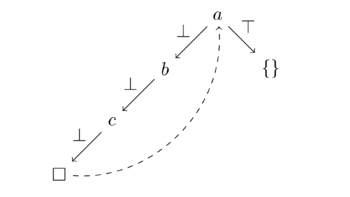Instead of backtracking to each previous variable, one can "jump" immediately to the cause of the contradictions.

#### Learning

Recall the previous example. Learning in CDCL is very simple: since assigning $a\equiv \bot$would lead to unsat, we simply conjunct the formula with the one-literal $a$thus ensuring the exclusion of that “branch” of the search. Explicitly, we obtain

$(a\lor b\lor c)\land (a\lor b\lor \lnot c)\land (a\lor \lnot b)\land a.$In the case that there are other clauses in the formula, say,

$(a\lor b\lor c)\land (a\lor b\lor \lnot c)\land (a\lor \lnot b)\land a\land \cdots$the inclusion of $a$as a one-literal clause will ensure its proper truth assignment and save the time spent in the wrong part of the search tree.

#### Performance

Since SAT remains $NP$-complete at the moment, any increases in performance that CDCL has over other implementations are unfortunately purely experimental. However, implementations with varying extents of backjumping and learning implemented saw significant increases in performance over simple DPLL implementations and some other then-modern implementations. The complexity of solving makes it necessary for hard cutoff times in the execution of the solver which upon reaching will result in failure. Under certain standardized test suites, simple DPLL implementations saw a 100% failure rate whereas CDCL implementations saw a 0% failure rate. Test suites were taken from a variety of sources to include planning, circuit diagnosis and synthesis, and scheduling instances.

The figure (right) contains an overview of CDCL on some of the test suites.

Bayardo Jr. et al. implemented four solvers based on DPLL and CDCL in C++. In the figure, naivesat is the DPLL implementation without backjumping or learning; cbjsat is DPLL with backjumping but without learning; sizesat is CDCL (ie. backjumping and learning) but only reasons with a bounded number of variables are retained; relsat is CDCL but only reasons with an upper bound on the number of variables with changed assignments since the reason was derived are retained.

For example, sizesat(2) would retain reasons such as $(a\lor \lnot b)$and $\lnot c$since these clauses have at most 2 variables. However, it will not retain reasons such as $(a\lor \lnot b\lor \lnot c)$.

On the other hand, relsat(2) will retain reasons such as $(a\lor b\lor c)$as long as $a$remains assigned to its original assignment (assuming that $a$, $b$, and $c$were assigned in the order they appear) because a reassignment of $a$would mean that both $b$and $c$have already been reassigned prior. The reassignment of $a$would then be the third change in assignment since the reason was derived and so then the reason is discarded.

### Conclusion

The official justification behind the research in "A Computing Procedure for Quantification Theory" and "A Machine Program for Theorem Proving" was the goal of automatically proving novel theorems mechanically. However, Davis later revealed that, together with Putnam, their primary motivation was for DPLL to be a source of funding for their work towards a more foundational and theoretical question in computability theory. It was unknown at the time that DPLL would lead to such widespread application. In the conclusion to their paper, Bayardo Jr. et al. cites not only planning, scheduling, circuit processing as systems in which DPLL appears, but also in more recent developments such as higher-order theorem provers or larger hybrid algorithms. Indeed, SAT solving techniques has inspired the closely related but more expressive SMT solvers which, loosely stated, combines SAT with first-order theories (eg. integer arithmetic, arrays, etc.). Moreover, DPLL has recently been further abstractified for the sake of compatibility with satisfiability modulo theories with the aim of theorem proving in mind.

On the other hand, the constant appearance of DPLL in contemporary systems, in a less positive light, suggests the lack of asymptotic improvements in the area. Despite the improved experimental performance in practice, it should be noted that CDCL is not significantly different from DPLL in that much of the original structure is maintained in the new algorithm. Progress usually attacks one of three places in the DPLL algorithm. Either novel techniques in backtracking are considered in the UnitPropagation section or different policies for choosing the branching variable are created or different data structures for storing the formula and clauses are introduced. CDCL falls into the first category.

The lack of an asymptotic speed-up for SAT since the 1960's is a testament to its complexity; the ubiquitous nature of SAT in not only automated reasoning but also theoretical computer science is a testament to its utility. It is wholly possible that, even if the event $P\neq NP$is realized, the search for more efficient procedures for SAT - experimental or otherwise - will continue.

## Annotated Bibliography

1. Marques-Silva, Joao (2008) Practical Applications of Boolean Satisfiability At Workshop on Discrete Event Systems (WODES'08), Sweden
2. Michael Sipser. 1996. Introduction to the Theory of Computation: Preliminary Edition (1st ed.). PWS Pub. Co., Boston, MA, USA.
3. Levin, Leonid (1973). "Универсальные задачи перебора,". Problems of Information Transmission (in Russian). 9 (3): 115–116.
4. Cook, Stephen (1971). "The complexity of theorem proving procedures". Proceedings of the Third Annual ACM Symposium on Theory of Computing. pp. 151–158. doi:10.1145/800157.805047
5. A. Biere, A. Biere, M. Heule, H. van Maaren, and T. Walsh. 2009. Handbook of Satisfiability: Volume 185 Frontiers in Artificial Intelligence and Applications. IOS Press, Amsterdam, The Netherlands, The Netherlands.
6. Davis, Martin; Putnam, Hilary (1960). "A Computing Procedure for Quantification Theory". Journal of the ACM. 7 (3): 201–215. doi:10.1145/321033.321034.
7. Davis, Martin; Logemann, George; Loveland, Donald (1962). "A Machine Program for Theorem Proving". Communications of the ACM. 5 (7): 394–397. doi:10.1145/368273.368557.
8. Hartmanis, J.; Stearns, R. E. "On the computational complexity of algorithms". Trans. Amer. Math. Soc. 117 1965 285–306.
9. J. P. Marques Silva and K. A. Sakallah, "GRASP-A new search algorithm for satisfiability," Proceedings of International Conference on Computer Aided Design, San Jose, CA, USA, 1996, pp. 220-227. doi: 10.1109/ICCAD.1996.569607
10. Roberto J. Bayardo Jr.; Robert C. Schrag (1997). "Using CSP look-back techniques to solve real world SAT instances". Proc. 14th Nat. Conf. on Artificial Intelligence (AAAI). pp. 203–208.
11. J. P. Marques-Silva and K. A. Sakallah, "GRASP: a search algorithm for propositional satisfiability," in IEEE Transactions on Computers, vol. 48, no. 5, pp. 506-521, May 1999. doi: 10.1109/12.769433
12. https://github.com/enzet/symbolic-execution/blob/master/diagram
13. Nieuwenhuis, Robert; Oliveras, Albert; Tinelli, Cesar (2004), "Abstract DPLL and Abstract DPLL Modulo Theories", Proceedings Int. Conf. on Logic for Programming, Artificial Intelligence, and Reasoning, LPAR 2004, pp. 36–50

## To Add

Formatting, Images, Diagrams, SourcesPermission is granted to copy, distribute and/or modify this document according to the terms in Creative Commons License, Attribution-NonCommercial-ShareAlike 3.0. The full text of this license may be found here: CC by-nc-sa 3.0